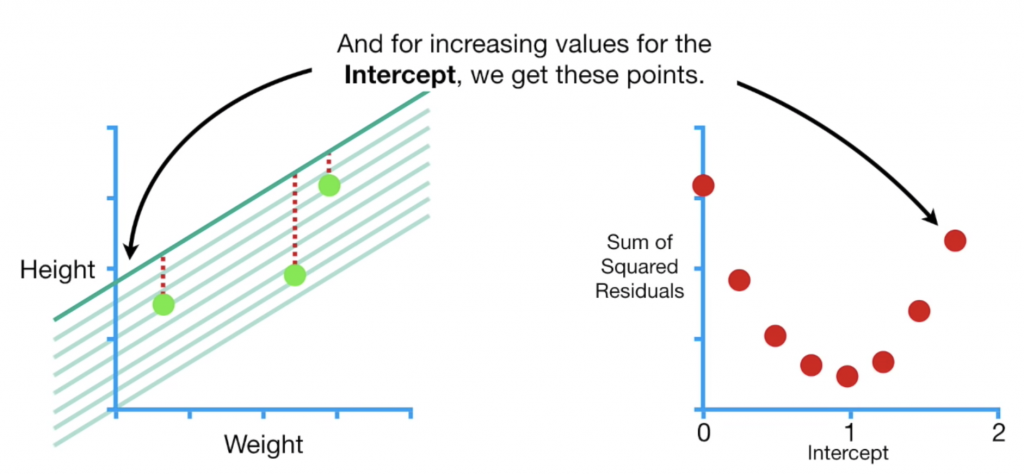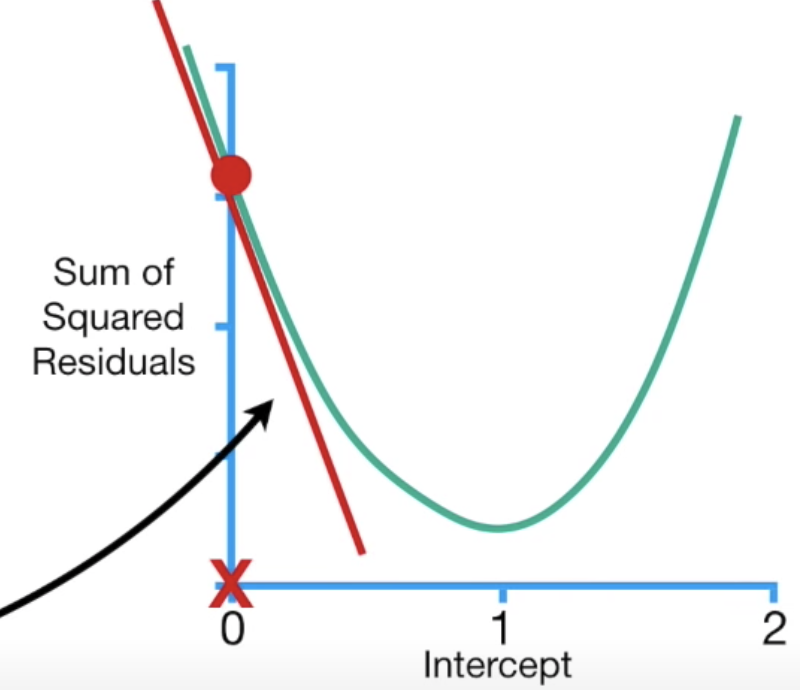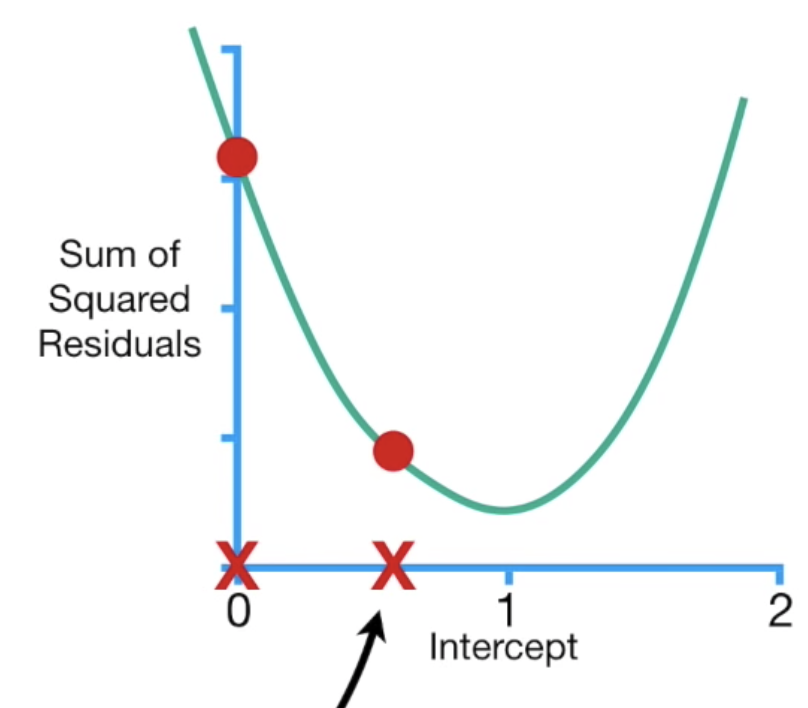#DAY 10
0
AI & Data

## 原理

2. 對參數隨機設置起始值：你可以說剛開始大家都是0，或是任何數字
4. 計算step: step=slope * learning rate
5. 計算新的參數值：等於舊的參數值-step
6. 重複步驟3-5直到你的slope/step趨近於0data: (0.5, 1.4),(2.3, 1.9),(2.9, 3.2)
SSR = (y - y_pred)^2 =
(1.4 - (0.64 * 0.5 + b))^2 +
(1.9 - (0.64 * 2.3 + b))^2 +
(3.2 - (0.64 * 2.9 + b))^2
= (1.08 - b)^2 + (0.42-b)^2 + (1.34-b)^2

= 2(1.08 - b)(-1) + 2(0.42 - b)(-1)+2(1.34 - b)*(-1)
= -2(1.08 - b + 0.42 - b + 1.34 - b) **
= -5.68 + 3b